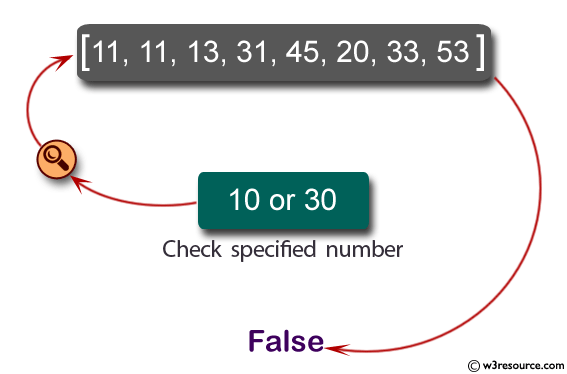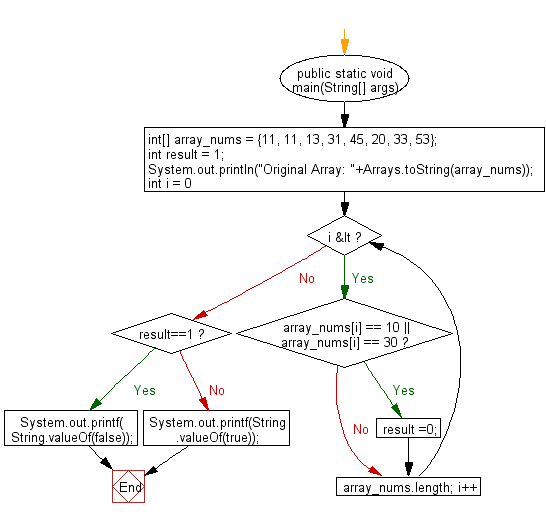﻿ Java: Check if an array of integers contains 10 or 30# Java Exercises: Check if a specified array of integers contains 10 or 30

## Java Basic: Exercise-102 with Solution

Write a Java program to check if a specified array of integers contains 10 or 30.

Pictorial Presentation:Sample Solution:

Java Code:

``````import java.util.*;
import java.io.*;
public class Exercise102 {
public static void main(String[] args)
{
int[] array_nums = {11, 11, 13, 31, 45, 20, 33, 53};
int result = 1;
System.out.println("Original Array: "+Arrays.toString(array_nums));

for(int i = 0; i < array_nums.length; i++)
{
if(array_nums[i] == 10 || array_nums[i] == 30)
{

result =0;
}
}
if (result==1)
System.out.printf( String.valueOf(false));
else
System.out.printf(String.valueOf(true));
}
}
```
```

Sample Output:

```Original Array: [11, 11, 13, 31, 45, 20, 33, 53]
false
```

Flowchart:Java Code Editor:

What is the difficulty level of this exercise?

Test your Programming skills with w3resource's quiz.

﻿

## Java: Tips of the Day

countOccurrences

Counts the occurrences of a value in an array.

Use Arrays.stream().filter().count() to count total number of values that equals the specified value.

```public static long countOccurrences(int[] numbers, int value) {
return Arrays.stream(numbers)
.filter(number -> number == value)
.count();
}
```

Ref: https://bit.ly/3kCAgLb